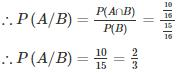Courses

# Engineering Mathematics 7

## 10 Questions MCQ Test RRB JE for Computer Science Engineering | Engineering Mathematics 7

Description
This mock test of Engineering Mathematics 7 for Computer Science Engineering (CSE) helps you for every Computer Science Engineering (CSE) entrance exam. This contains 10 Multiple Choice Questions for Computer Science Engineering (CSE) Engineering Mathematics 7 (mcq) to study with solutions a complete question bank. The solved questions answers in this Engineering Mathematics 7 quiz give you a good mix of easy questions and tough questions. Computer Science Engineering (CSE) students definitely take this Engineering Mathematics 7 exercise for a better result in the exam. You can find other Engineering Mathematics 7 extra questions, long questions & short questions for Computer Science Engineering (CSE) on EduRev as well by searching above.
QUESTION: 1

###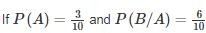where A and B are exhaustive events, then find P(B)?

Solution:

Since A and B are exhaustive events

∴ P(A∪B) = 1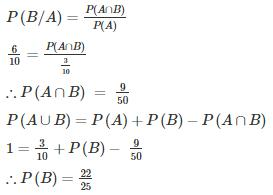QUESTION: 2

### In an examination, 20% of the students have failed in Computer Networks, 30% of the students have failed in Computer Architecture and 10% in Computer Networks and Computer Architecture. If a student is selected at random, then what is the probability that the student has failed in at least one subject?

Solution:

Let the number of students be 100.

P(N)→ probability that students failed in Computer Networks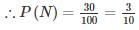P(A)→ probability that students failed in Computer Architecture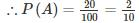P(N∩A)→ probability that the student has failed in both the subject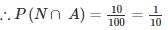P(NUA)→ probability that the  student has failed in atleast one subject

P(NUA) = P(N) + P(A) − P(N∩A)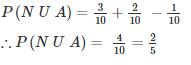QUESTION: 3

### A coin is tossed 9 times. What is the probability that the outcome of only first two tosses is headed?

Solution:

Required probability =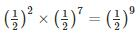QUESTION: 4

A pair of dice is thrown. Find the probability of obtaining a sum of 8 or getting an even number on both the dice.

Solution:

Let the events be defined as:

A: Obtaining a sum of 8

B: Getting an even number on both dice

P(A U B) = P(A) + P(B) – P (A ∩ B)

Now cases favourable to A are (3, 5) (5, 3) (2, 6) (6, 2) (4, 4)

So, P(A) = 5/36

Cases favourable to B: (2, 2), (2, 4), (2, 6), (4, 2), (4, 4), (4, 6), (6, 2), (6, 2), (6, 4), (6, 6).

P(B) = 9/36

Now, (2, 6) (6, 2) and (4, 4) are common to both events A and B

So, P (A ∩ B) = 3/36

⇒ P (A ∪ B) = (5/36) + (9/36) – (3/36) = 11/36

QUESTION: 5

A bag contains 6 red, 5 blue balls, and another bag contains 5 red and 8 blue balls. A ball is drawn from the first bag without noticing the color is put in the second bag. A ball is drawn from the second bag. Find the probability that ball drawn is blue in color.

Solution:

P(A)→ probability of getting a blue ball from the 2nd bag.

P(E1)→ probability of getting a red ball from the 1st bag.

P(E2)→ probability of getting a blue ball from the 1st bag.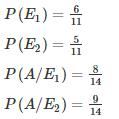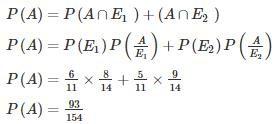*Answer can only contain numeric values
QUESTION: 6

The chances of A, B, C becoming managers of a Testbook company are 4:2:3. The probability that the bonus scheme will be introduced if A, B, C become manager are 0.3, 0.5, 0.8 respectively.

If the bonus scheme has introduced, what is the probability that A is appointed as the manager of Testbook (answer up to 2 decimal place)?

Solution:

P(X)→ probability that bonus scheme is introduced

P(A) → probability of A being a manager.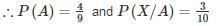P(B) → probability of B being a manager.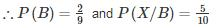P(C) → probability of C being a manager.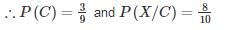P(X) = P (A ∩ X) + P (B ∩ X) + P (C ∩ X)

P(X) = P(A)P(X/A) + P(B)P(X/B) + P(C)P(X/C)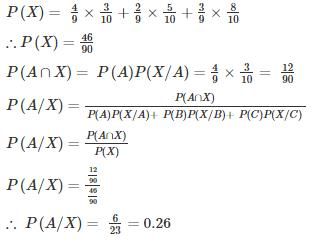*Answer can only contain numeric values
QUESTION: 7

A speaks truth 3 out of 4 times. There is a chance that match can be won, drawn or lost but A reported that Shyam has won the match. Find the probability that his report was correct.

Solution:

T: A speaks truth ⇒ P(T) = ¾

T̅ : A lies ⇒ P(T̅) = 1 - P(T) = ¼

There are three cases for matches. It can be won, drawn or lost.

The probability of winning a match, P (B/T) = 1/3

The probability of not winning a match, P(B,T̅) = 2/3

Using Baye’s theorem: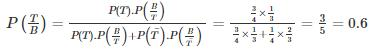*Answer can only contain numeric values
QUESTION: 8

A and B throw a pair of dice alternately. A wins if he throws faces with sum 5 before B throws faces with the sum of 6 and B wins if he throws faces with the sum of 6 before A throw faces with the sum of 5. If A begins, the chances of winning for A is________.

Solution:

The sum 5 can be obtained as follows:

(1, 4), (2, 3), (3, 2), (4, 1)

The probability of A is throwing 5 with two dice is

= 4/36 = 1/9

The probability of A is not throwing 5 is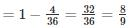The sum 6 can be obtained as follows:

(1, 5), (2, 4), (3, 3), (4, 2), (5, 1)

The probability of B is throwing 6 is

= 5/36

The probability of B is not throwing 6 is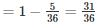Given that A begins,

Now A can win if he throws 5, in first chance,

(or) third Chace (b) fifth chance etc –

The chances of winning of A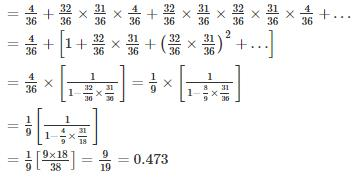*Answer can only contain numeric values
QUESTION: 9

An anti-aircraft gun can take a maximum of 4 shots at an enemy plane moving away from it. The probabilities of hitting the plane at the first, second, third and fourth shot are 0.4, 0.3, 0.2 and 0.1 respectively. The probability that the gun hits the plane is_____

Solution:

Let p1 = 0.4, p2 = 0.3, p3 = 0.2 and p4 = 0.1

P(the gun hits the plane) = P(the plane is hit at least once)

= 1 – P(the plane is hit in none of the shots)

= 1− (1−p1) (1−p2) (1−p3) (1−p4)
= 1− (0.6 × 0.7 × 0.8 × 0.9)
= (1− 0.3024) = 0.6976

QUESTION: 10

What is the probability of getting more than one tail given that at least one coin is head when 4 coins are tossed?

Solution:

P(A)→ probability of getting more than one tail

∴ P(A) = 11/16

P(B)→ probability of getting at least one tail

∴ P(B) = 15/16

P(A ∩ B)→ probability of getting more than one tail and at least one is head.

∴ P(A ∩ B) = 10/16